Unlimited PowerPoint templates, graphics, videos & courses! Unlimited asset downloads! From \$16.50/m
Advertisement# How to Calculate Percentages in Excel With Formulas

Difficulty:IntermediateLength:LongLanguages:

If you need to work with percentages, you’ll be happy to know that Excel has tools to make your life easier.

You can use Excel to calculate percentage increases or decreases to track your business results each month. Whether it’s rising costs or percentage sales changes from month to month, you want to keep on top of your key business figures. Excel can help you do that.

You’ll also learn how to work with advanced percentage calculations using the scenario of calculating grade point averages, as well as discover how to figure out percentile rankings, which are both relatable examples that you can apply to a variety of use cases.

In this tutorial, learn how to calculate percentages in Excel with step-by-step workflows. Let’s look at some Excel percentage formulas, functions, and tips using a sheet of business expenses and a sheet of school grades.

You’ll walk away with the techniques needed to work proficiently with percentages in Excel.

## Screencast

Watch the complete tutorial screencast, or work through the step-by-step written version below. First, download the source files for free: Excel percentages worksheets. We'll use them to work through the tutorial exercises.

## 1. Input Initial Data in Excel

Input the data as follows (or start with the download file "percentages.xlsx" contained in the tutorial source files). This worksheet is for Expenses. Later in this tutorial, we’ll use the Grades worksheet.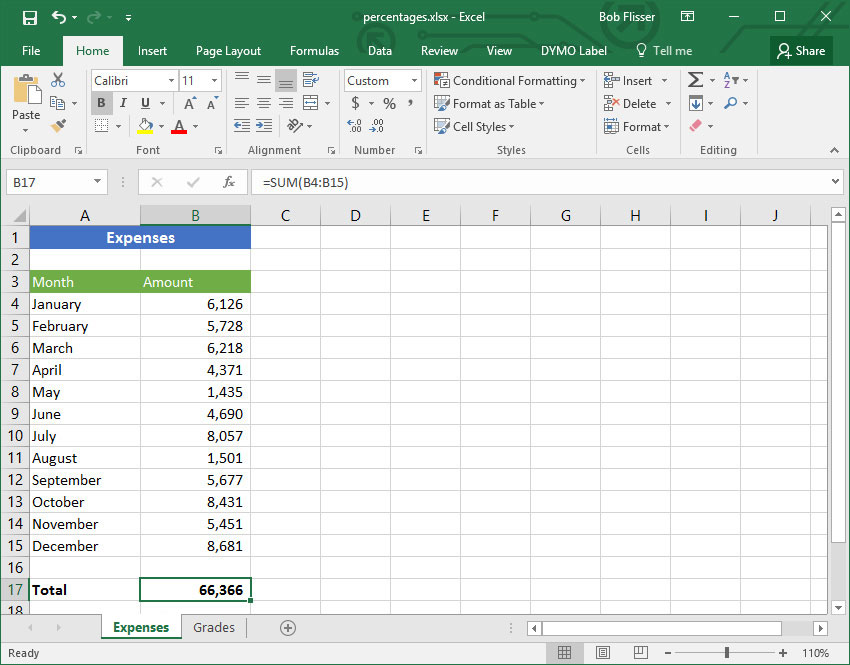Excel percentages worksheet data

## 2. Calculate a Percentage Increase

Let’s say you anticipate that next year’s costs will be 8% higher, so you want to see what they are.

Before writing any formulas, it’s helpful to know that Excel is flexible enough to calculate the same way whether you type percentages with a percent sign (like 20%) or as a decimal (like 0.2 or just .2). To Excel, the percent symbol is just formatting.

We want to show the total estimated amount, not just the increase.

### Step 1

In A18, type the header With 8% increase. Since we have a number mixed with text, Excel will treat the entire cell as text.

### Step 2

Press Tab, then in B18, enter this Excel percentage formula: `=B17 * 1.08`

Alternatively, you can enter the formula this way: `=B17 * 108%`

The amount is 71,675, as shown below: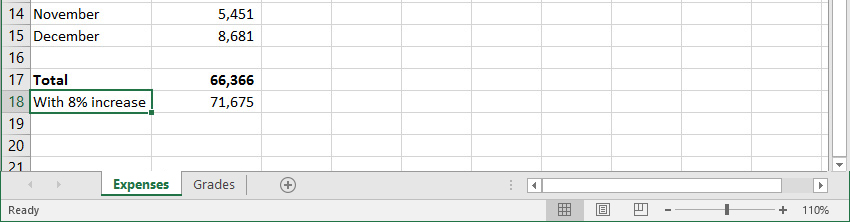Calculating a percentage increase in Excel

## 3. Calculate a Percentage Decrease

Maybe you think your expenses will decrease by 8 percent instead. To see those numbers, the formula is similar. Start by showing the total, lower amount, not just the decrease.

### Step 1

In A19, type the header With 8% decrease.

### Step 2

Press Tab, then in B19, enter this percentage formula in Excel: `=B17 * .92`

Alternatively, you can enter the formula this way: `=B17 * 92%`

The amount is 61,057.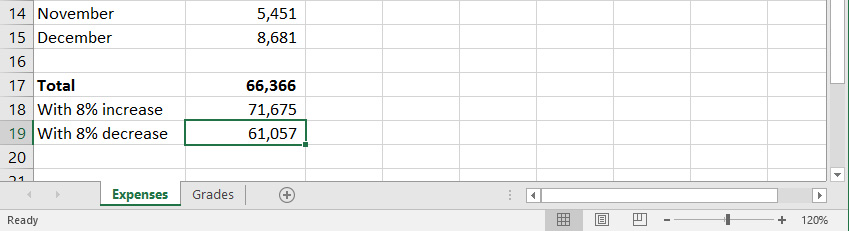Calculating a percentage decrease in Excel

### Step 3

If you want a little more flexibility in changing the percentage by which you think the costs will rise or drop, you can enter the formulas this way, instead:

For the 8% increase, enter this formula in B18`=B17 + B17 * 0.08`

### Step 4

For the 8% decrease, enter this Excel percentage formula in B19`=B17 – B17 * 0.08`

With these formulas, you can simply change the .08 to another number to get a new result from a different percentage.

## 4. Calculate a Percentage Amount

Now to work through an Excel formula for a percentage amount. What if you want to see the 8% amount itself, not the new total? To do that, multiply the total amount in B17 by 8 percent.

### Step 1

In A20, enter the header 8% of total.

### Step 2

Press Tab, then in B20 enter the formula: `=B17 * 0.08`

Alternatively, you can enter the formula this way: `=B17 * 8%`

The amount is 5,309.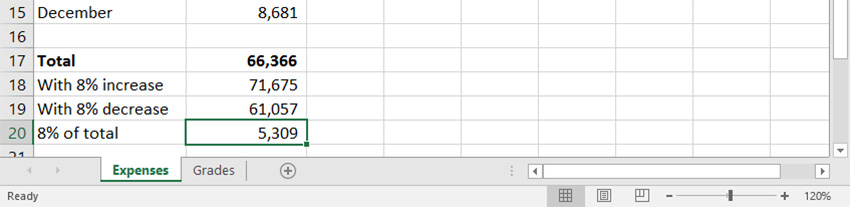Calculate a percentage total in Excel

## 5. Make Adjustments Without Rewriting Formulas

If you want to change the percentage without having to rewrite the formulas, put the percentage in its own cell. We’ll start by entering row titles.

### Step 1

In A22, type Adjustment. Press Enter.

### Step 2

In A23, type Higher total. Press Enter.

### Step 3

In A24, type Lower total. Press Enter.

The worksheet should now look like this: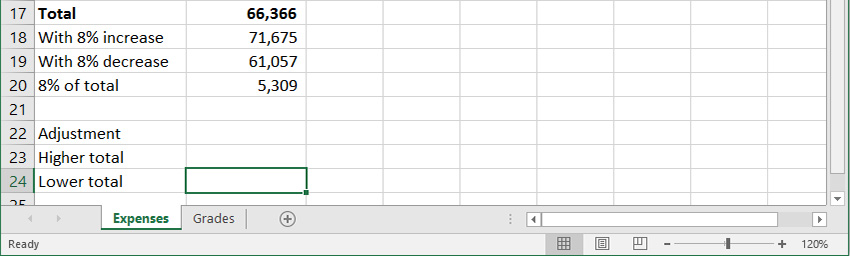Excel sheet with additional row titles

Now enter the percentage and the formulas to the right of the titles.

### Step 4

In B22, type 8%. Press Enter.

### Step 5

In B23, enter this formula to give you the total plus another 8%: `=B17 + B17 * B22`

### Step 6

In B24, enter this formula to give you the total less 8%: `=B17 * (100% - B22)`

When you type the 8% in B22, Excel automatically formats the cell as a percentage. If you type .08 or 0.08, Excel will leave it like that. You can always format it as a percent later on by clicking the Percent Style button on the Ribbon: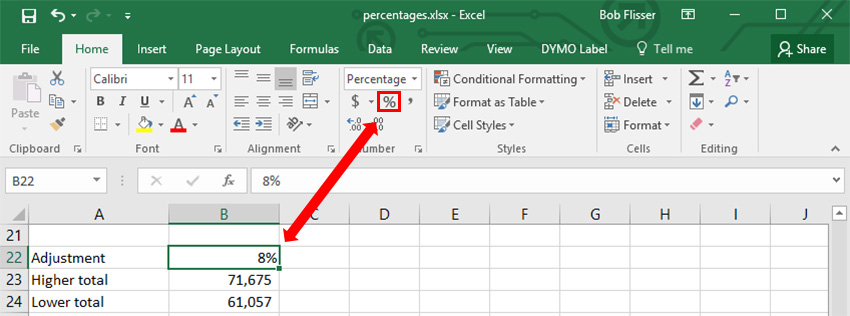Excel Percent Style button and its effect

Tip: you can also format the numbers as Percent Style using a keyboard shortcut: press Control-Shift-% in Windows or Command-Shift-% on the Mac.

## 6. Calculate a Percentage Change

You might also want to calculate the percentage change from one month to the next month. That would give you a picture of whether costs were heading up or heading down. So let’s do that down column C.

The general rule to calculate a percentage change is:

=(new value - old value) / new value

Since January is the first month, it doesn’t have a percentage change. The first change will be from January to February, and we’ll put this next to February’s number.

### Step 1

To calculate the first percentage change, enter this percent change formula in C5`=(B5-B4)/B5`

### Step 2

Excel displays this as a decimal, so click the Percent Style button on the Ribbon (or use the above mentioned shortcuts) to format it as a percent.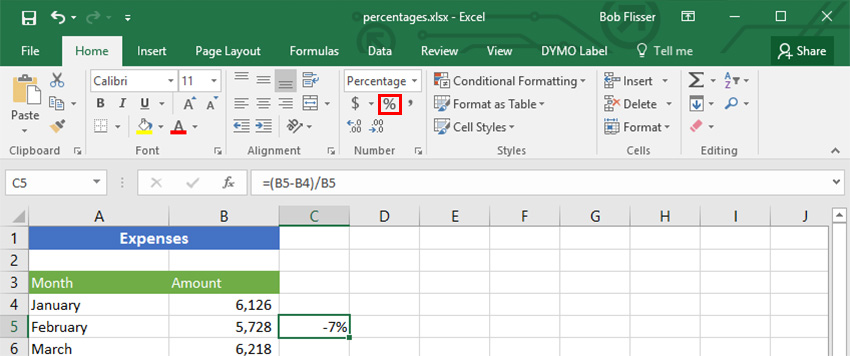Excel Percent Style button for percent changes

Now that we know what the percent change is from January to February, we can AutoFill the formula down column C to show the remaining percent changes for the year.

### Step 3

Roll the mouse pointer over the dot in the lower-right corner of the cell that shows -7%.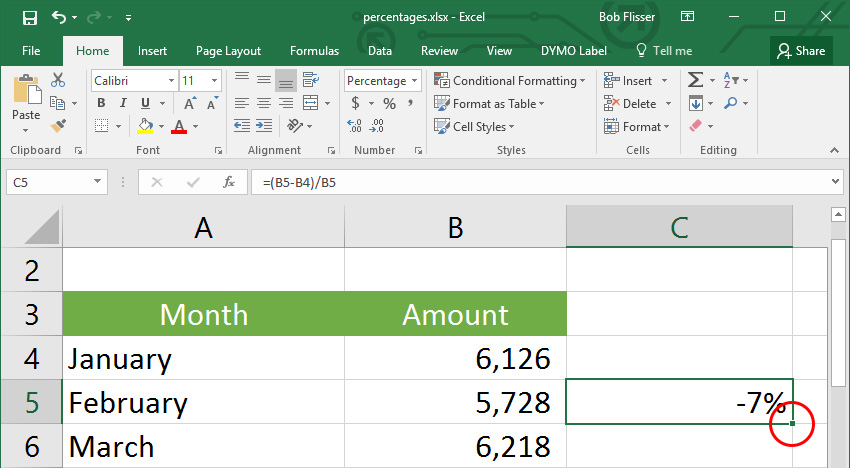Excel AutoFill dot

### Step 4

When the mouse pointer becomes a crosshair, Double-click.

If you’re unfamiliar with the AutoFill feature, see technique 3 in my Excel power techniques article:

### Step 5

Click cell B3 (the “Amount” header).

### Step 6

Put the mouse pointer on its AutoFill dot and drag one cell to the right, into C3. That duplicates the header, including the formatting.

### Step 7

In C3, type % Change, replacing the text that’s already there.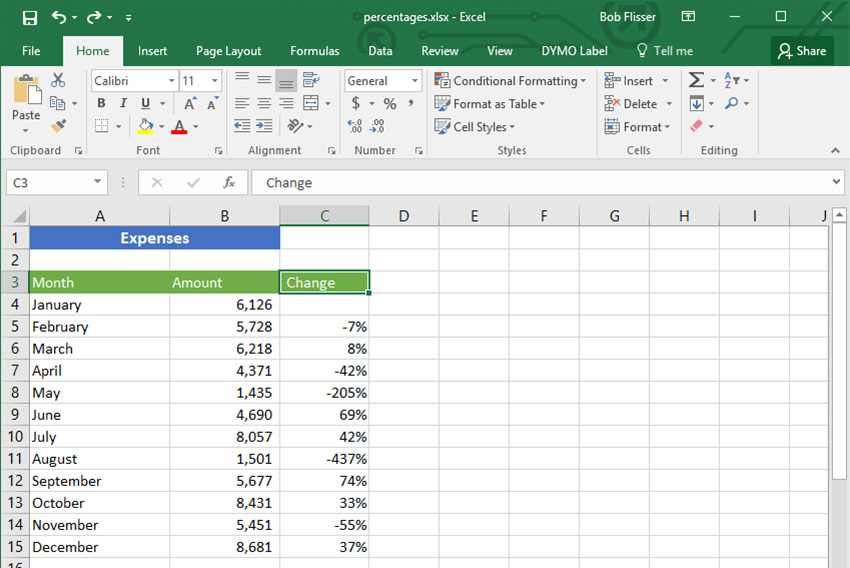All percent changes calculated

## 7. Calculate a Percentage of Total

The final technique on this sheet is to find the percent of total for each month. For a percent of total calculation, think of a pie chart where each month is a slice, and all the slices add up to 100%.

Whether with Excel or with pencil and paper, the way to calculate a percentage of total is with a simple division:

Component number/total

… and format it as a percentage.

In this example, we divide each month by the total at the bottom of column B.

### Step 1

Click on C3 and AutoFill one cell across to D3.

### Step 2

Change D3 to % of Total.

### Step 3

In D4, type this formula, but don’t press Enter, yet`=B4/B17`

### Step 4

Before entering the formula, we want to be sure of preventing AutoFill errors. Since we’re going to AutoFill down the column, the denominator, which is now B17, shouldn’t change. If it does, the numbers for February through December will be wrong.

Make sure the text cursor is still in the formula, on the “B17” denominator.

### Step 5

Press the F4 key (on the Mac, press Fn-F4).

That inserts dollar signs before the column and the row, turning the denominator into \$B\$17. The \$B means that column B won’t increase to column C, etc. and the \$17 means the row won’t increase to \$18, etc.

### Step 6

Make sure the percentage formula in excel is now: `=B4/\$B\$17`.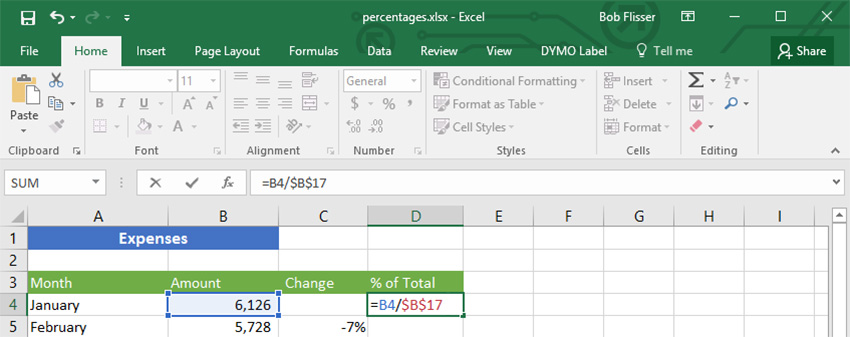Percent of total formula

### Step 7

Now we can enter and AutoFill.

Press Control-Enter (on the Mac, press Command-↩) to enter the formula without moving the cursor down to the next row.

### Step 8

Click the Percent Style button on the ribbon or use the Control-Shift-% (Command-Shift-%) shortcut.

### Step 9

Roll the mouse pointer over the AutoFill dot in the lower-right corner of D4.

### Step 10

When the cursor becomes a crosshair, double-click to AutoFill the formula down to the bottom.

Each month will now show how much of a percentage of the grand total it is.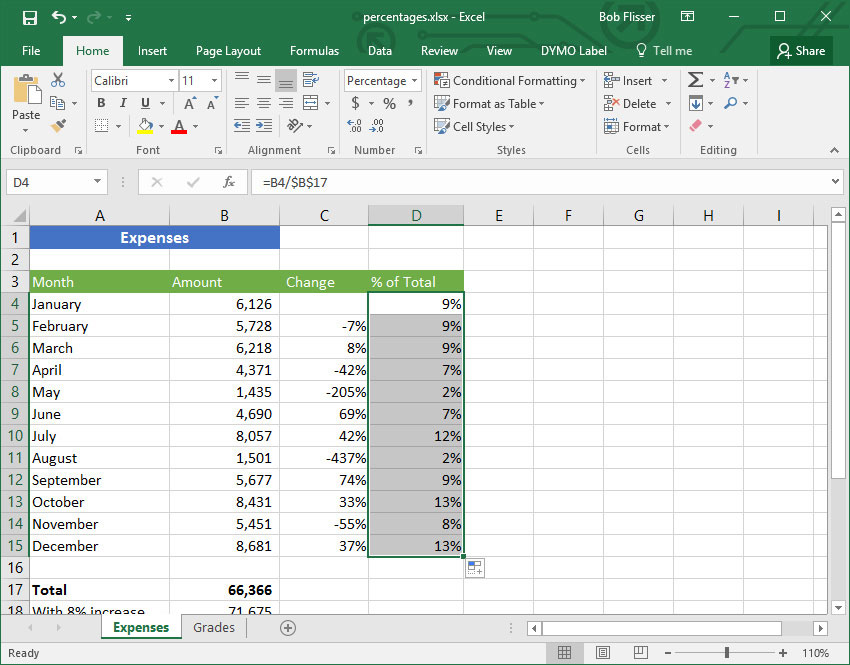Percent of totals filled in

## 8. Percentage Ranking

Ranking numbers by percent is a statistical technique. You’re probably familiar with it from school: you and your classmates have grade point averages, so each of you is ranked in a percentile. The higher your grades, the higher your percentile.

The list of numbers (grades, in this example) are in a range of cells that Excel calls an array. There’s nothing special about an array and you don’t have to define it. That’s just what Excel calls the range of cells you plug into a formula.

Excel has two functions for percentage ranking. One function includes the beginning and ending numbers of the array and the other function doesn’t.

Look at the second tab in the worksheet: Grades.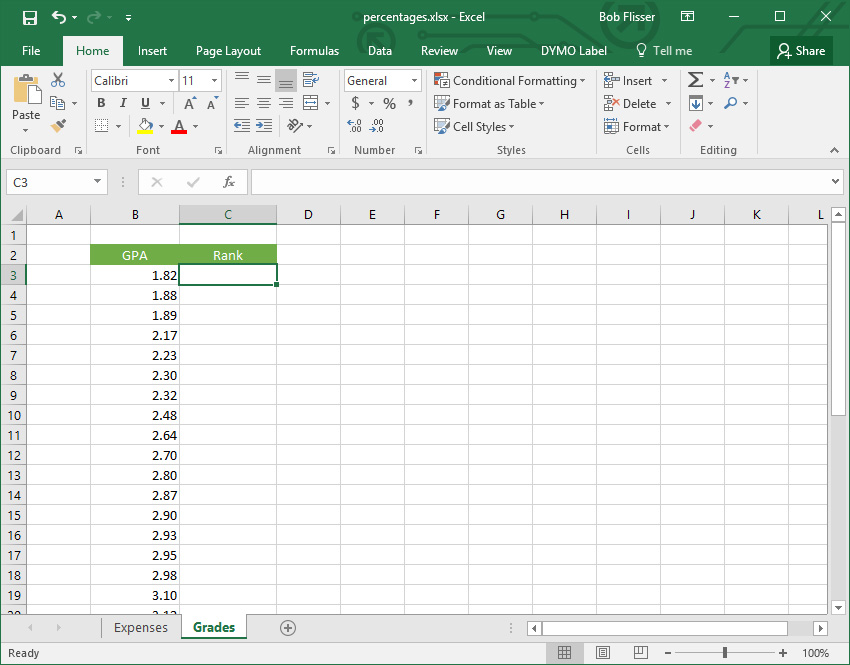Excel Percent ranking - Grades worksheet

This shows a list of 35 grade point averages, sorted in ascending order. The first thing we want to do is find the percentile rank for each one. To do this, we’ll use the =PERCENTRANK.INC function. The “INC” part of that function means “inclusive," as it will include the first and last grades in the list. If you want to exclude the first and last numbers in the array, you would use the =PERCENTRANK.EXC function.

The function takes two mandatory arguments and the syntax is: ```=PERCENTRANK.INC(array, entry)```

• array is the range of cells that contains the list (in this example, it’s B3:B37)
• entry is any number or cell in the list

### Step 1

Click C3, the first one in the list.

### Step 2

Start typing this formula, but don’t press Enter, yet`=PERCENTRANK.INC(B3:B37`

### Step 3

This range needs to be an absolute reference so we can AutoFill to the bottom.

Press F4 (on the Mac, press Fn-F4) to insert dollar signs.

The formula should now look like this: `=PERCENTRANK.INC(\$B\$3:\$B\$37`

### Step 4

In each case down column C, we want to know the percent rank of the entry in column B.

Click B3, then close the parenthesis.

The formula is now this: `=PERCENTRANK.INC(\$B\$3:\$B\$37,B3)`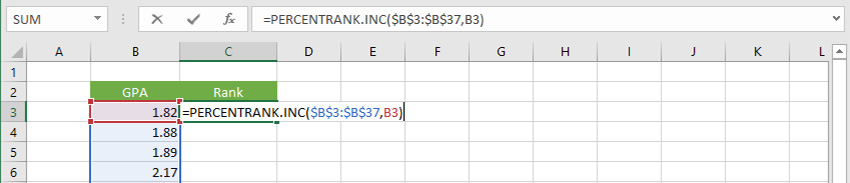Excel Percentrank function

Now we can fill in and format the numbers.

### Step 5

If necessary, click back on C3 to select it.

### Step 6

Double-click the AutoFill dot on C3. That automatically fills down the column.

### Step 7

On the Ribbon, click the Percent Style button to format the column as percentages. You should now see the finished result: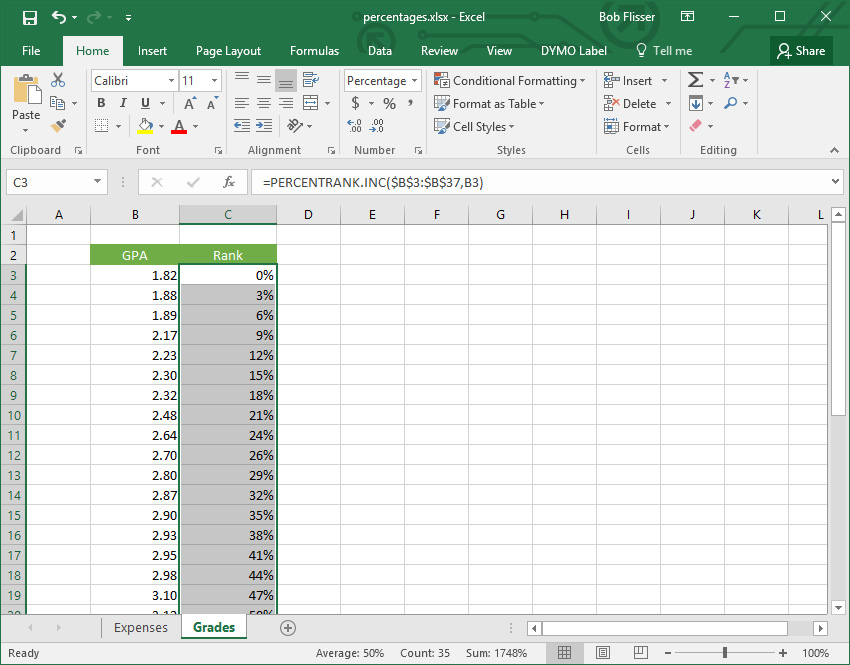Excel Percentrank function result filled and formatted

So if you had very good grades and your GPA was 3.98, you would say that you ranked in the 94th percentile.

## 9. Finding the Percentile

You can also find the percentile directly, using the PERCENTILE functions. With these functions, you enter a percent rank, and it will return a number from the array that corresponds to that rank. If the exact number you want isn’t listed, Excel will interpolate the result and return the number that “should” be there.

When would you use this? Let’s say you plan on applying for a graduate program, and the program accepts only students who score in the 60th percentile or higher. So you want to know what GPA is exactly 60%. Looking at this list, we can see 3.22 is at 59% and 3.25 is at 62%, so we can use the =PERCENTILE.INC function to find the answer.

The syntax is: =PERCENTILE.INC(array, percent rank)

• array is the range of cells that contains the list (same as the previous example, B3:B37)
• rank is a percentage (or a decimal between and including 0 and 1)

Just like with the percent ranking functions, you could use =PERCENTILE.EXC to exclude the first and last entries in the array, but that’s not what we want in this example.

### Step 1

Click in B39, below the list.

### Step 2

Enter this formula: `=PERCENTILE.INC(B3:B37,60%)`

Since we aren’t going to AutoFill this formula, there is no need to make the array an absolute reference.

### Step 3

On the Ribbon, click the Decrease Decimal button once, to round the number to two decimal places.

The result is that in this array, a 3.23 GPA is the 60th percentile. Now you know the grades you need for acceptance into the program.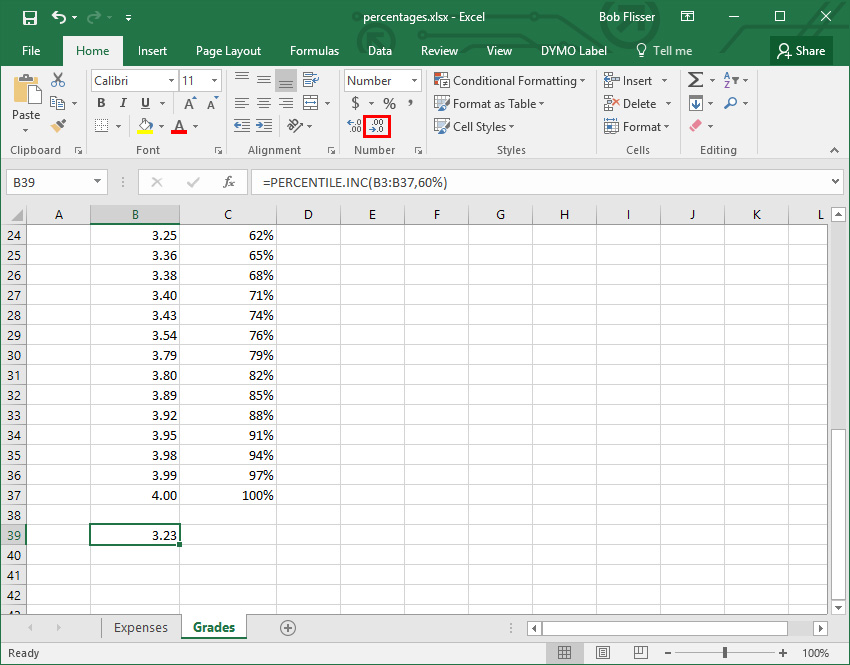Percentile function result

## Conclusion

Percentages aren’t complicated, and Excel calculates them using the same rules of math as you would use with pencil and paper. Excel also adheres to the standard order of operations when you have addition, subtraction, and multiplication in a formula:

1. Parentheses
2. Exponents
3. Multiplication
4. Division
5. Addition
6. Subtraction

I always remember this with the mnemonic Please Excuse My Dear Aunt Sally.

Advertisement
Advertisement
Advertisement